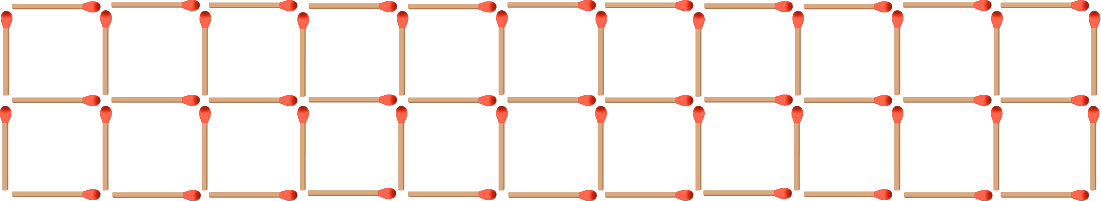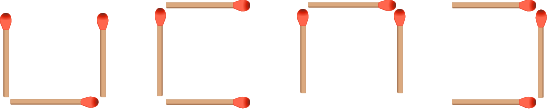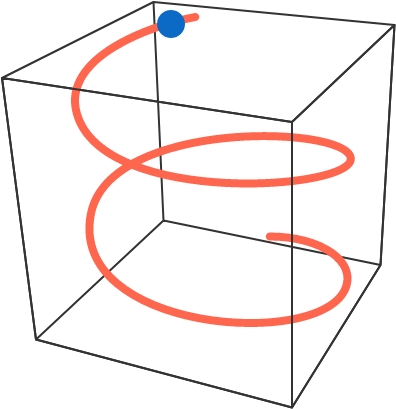# 2017-08-21 Advanced

The image below is made up of 57 matchsticks:Define a move to be removing 3 matchsticks in any of the following shapes:What is the largest number of successive moves that can be made?

All points on a Cartesian plane are colored either $\color{#D61F06}{\text{red}}$ or $\color{#3D99F6}{\text{blue}}$.

For all positive numbers $r,$ there exists a non-degenerate isosceles triangle with legs of length $r$ whose vertices are all colored $\text{\_\_\_\_\_\_\_\_\_\_}$.

Is the statement above true for at least one of the colors?


Note: A degenerate triangle is one where all three vertices lie on a straight line.

A bead slides from rest down a wire that's bent into a helix, which can be parametrized in the following way: $\begin{cases} x = \cos(\theta) \\ y = \sin(\theta) \\ z = \theta. \end{cases}$ Find the magnitude of the bead's vertical acceleration.Assumptions: The bead slides without friction and there is a uniform, gravitational field $-g\,\hat{\mathbf{z}}.$

$\large{T_m=\underbrace{17^{17^{\cdot^{\cdot^{\cdot^{17}}}}}}_{m \text{ times}}}$

Find the smallest possible value of $n$ such that the numbers $T_n,T_{n+1},T_{n+2},\ldots$ all have the same last (rightmost) 2017 digits.

Bonus: Generalize to the last $k$ digits.

You have an infinite stream of digits from 0-9, each digit produced uniformly at random. You want to produce a random integer in the following, unusual manner:

First, take the first digit of the stream. Then, for each of the next digits, if it's larger than the last digit you took, ignore it. Otherwise, take it as well. This process ends when you take a 0 (since everything else will be 0). Your number is the sum of all the numbers taken.

For example, consider the following stream: 573493205714390...

1. First, you take the 5.
2. The next digit is a 7, so you ignore it.
3. The next digit is a 3, so you take it. Now the last digit you took is 3.
4. The next digit is a 4. Despite this being less than 5, you ignore it, since the last digit you took is 3 and 4 is larger than 3.
5. The next digit is a 9, which is also skipped.
6. The next digit is a 3. This is taken since you only skip strictly larger numbers.
7. The next digit is a 2, which is also taken.
8. The next digit is a 0, which ends the process since you're not going to take any other digit than 0. The resulting number produced by your process is $5+3+3+2 = 13$.

This problem has two parts, both relating to this generator; you need to solve both of them.

1. It can be proven that not only the process will end, the expected number you'll get is also finite and a rational number. The expected number you get can be represented as $\frac{a}{b}$ with $a,b$ positive integers that are relatively prime.
2. There exists a smallest integer $N$ such that 99.99% of the time, the obtained number is strictly smaller than $N$ $($and the obtained number is smaller than $N-1$ less than 99.99% of the time$).$

Find $10^6 a + 10^3 b + N$.

×

Problem Loading...

Note Loading...

Set Loading...